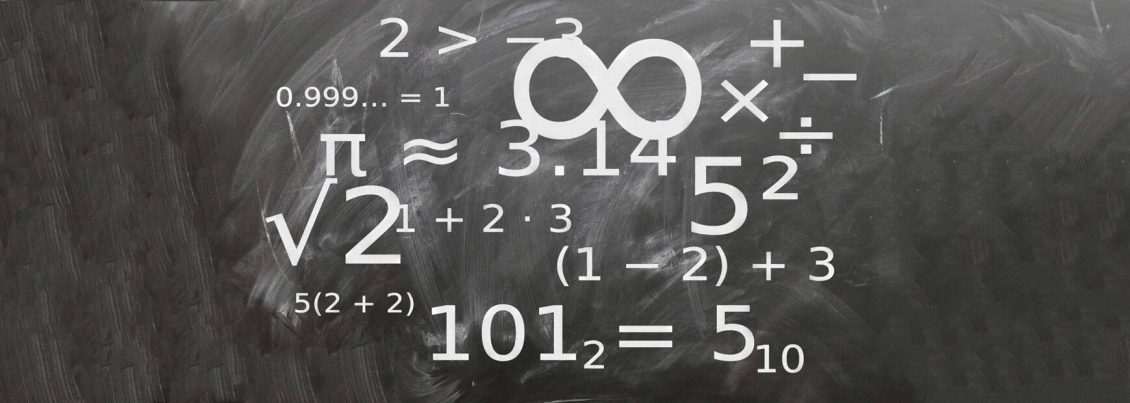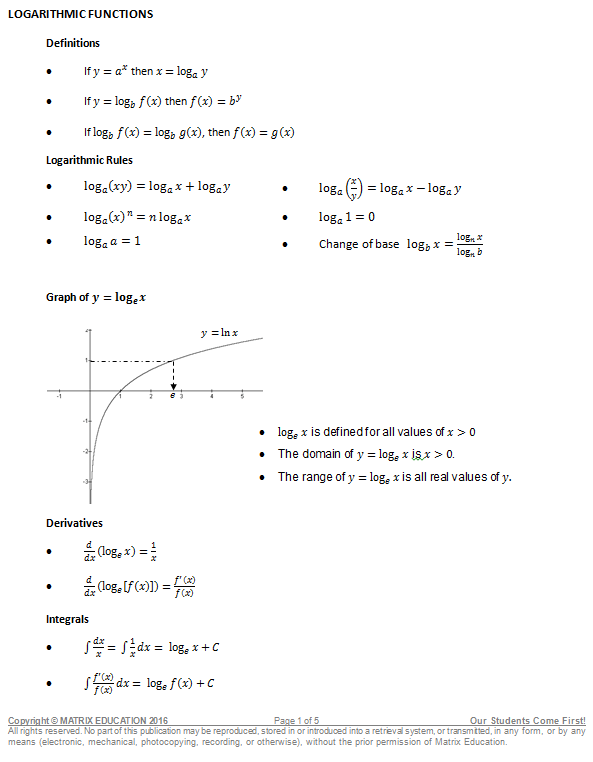The Ultimate Maths ADV Exponentials and Logarithms Summary SheetExponential and logarithmic functions are important in both theory and practice, and have many real-life applications pertaining to the mathematics, science and engineering fields. This Mathematics Advanced Summary Sheet includes a review of the key information regarding exponentials and logarithms – the definitions, rules, graphs, derivatives and integrals of each. Test your knowledge by answering the two exponentials and logarithms questions included in the summary sheet.

Preview: